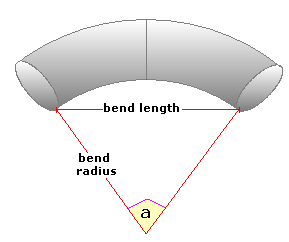Spike's Calculators

# Pipe Bend Radius Calculated from an AngleCalculate a pipe's bend radius using the curve's internal angle and the bend's length. This calculator uses inches for measurements.

Internal Angle in
Length of Curve in
Decimal Precision #

#### Calculator

1. enter the angle of the curve in degrees
2. the length of the curve in inches
3. decimal precision, number of digits after the decimal point

#### Results

1. the bend radius in inches

##### Formula
```r = 0.5L/sin(a/2)
where r is the bend radius
L the bend length
the internal angle of the curve
```